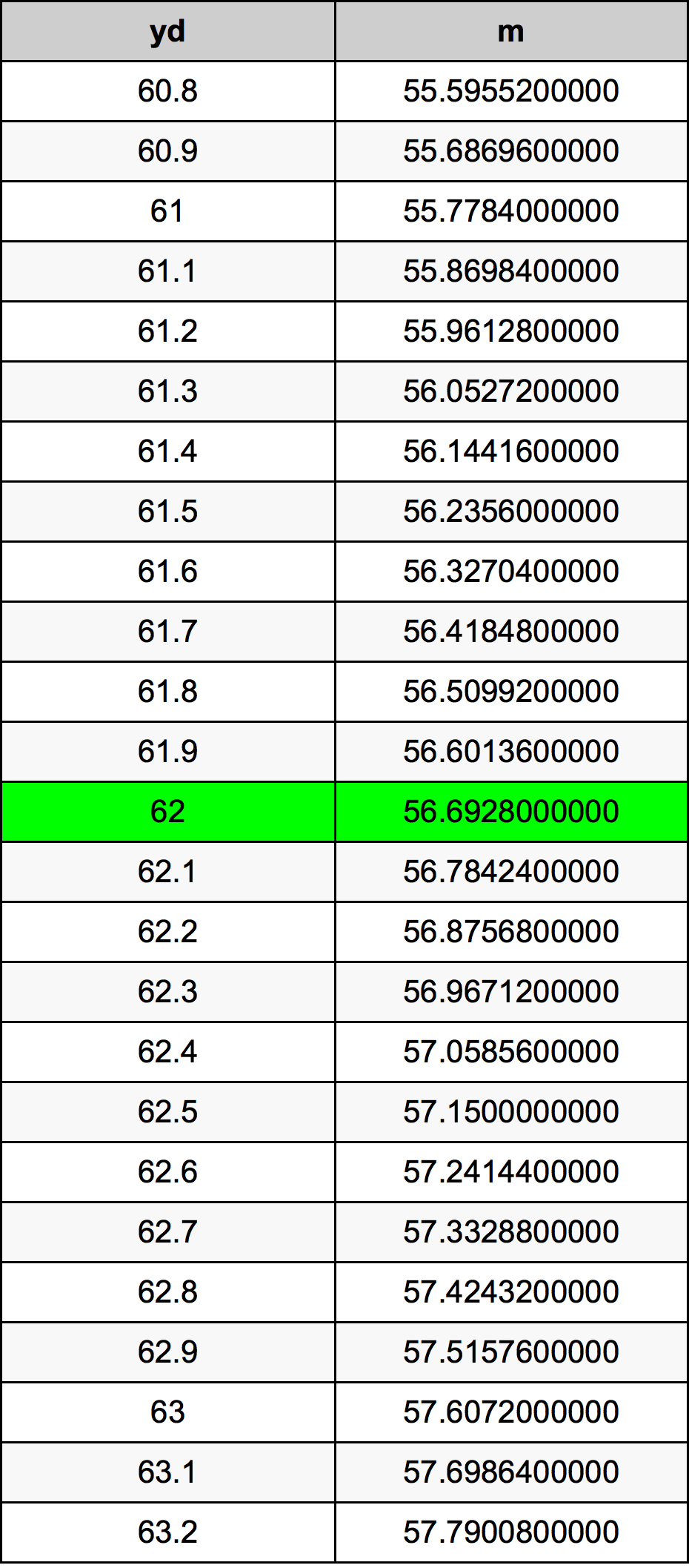Yards To Meters

# 62 yd to m62 Yards to Meters

yd
=
m

## How to convert 62 yards to meters?

 62 yd * 0.9144 m = 56.6928 m 1 yd
A common question is How many yard in 62 meter? And the answer is 67.8040244969 yd in 62 m. Likewise the question how many meter in 62 yard has the answer of 56.6928 m in 62 yd.

## How much are 62 yards in meters?

62 yards equal 56.6928 meters (62yd = 56.6928m). Converting 62 yd to m is easy. Simply use our calculator above, or apply the formula to change the length 62 yd to m.

## Convert 62 yd to common lengths

UnitLength
Nanometer56692800000.0 nm
Micrometer56692800.0 µm
Millimeter56692.8 mm
Centimeter5669.28 cm
Inch2232.0 in
Foot186.0 ft
Yard62.0 yd
Meter56.6928 m
Kilometer0.0566928 km
Mile0.0352272727 mi
Nautical mile0.0306116631 nmi

## What is 62 yards in m?

To convert 62 yd to m multiply the length in yards by 0.9144. The 62 yd in m formula is [m] = 62 * 0.9144. Thus, for 62 yards in meter we get 56.6928 m.

## 62 Yard Conversion Table## Alternative spelling

62 yd to m, 62 yd in m, 62 yd to Meters, 62 yd in Meters, 62 yd to Meter, 62 yd in Meter, 62 Yard to Meter, 62 Yard in Meter, 62 Yard to m, 62 Yard in m, 62 Yard to Meters, 62 Yard in Meters, 62 Yards to Meters, 62 Yards in Meters